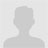• 二次方程的标准形式为：ax2+bx+c=0，其中a，b和c是实数，a≠0该b2-4ac称为二次方程的行列式。行列式说明了的性质。如果行列式大于0，则是实数且不同。如果行列式等于0，则是实数且相等。如果行列式小于0，则...
Java程序来查找二次方程式的所有根在此程序中，您将学习查找二次方程式的所有根，并使用Java中的format()打印它们。二次方程的标准形式为：ax2 + bx + c = 0，其中a，b和c是实数，a ≠ 0该b2-4ac称为二次方程的行列式。行列式说明了根的性质。如果行列式大于0，则根是实数且不同。如果行列式等于0，则根是实数且相等。如果行列式小于0，则根是复数且不同的。示例：查找二次方程式根的Java程序public class Quadratic {public static void main(String[] args) {double a = 2.3, b = 4, c = 5.6;double root1, root2;double determinant = b * b - 4 * a * c;//实数不同根的条件if(determinant > 0) {root1 = (-b + Math.sqrt(determinant)) / (2 * a);root2 = (-b - Math.sqrt(determinant)) / (2 * a);System.out.format("root1 = %.2f and root2 = %.2f", root1 , root2);}//实等根的条件else if(determinant == 0) {root1 = root2 = -b / (2 * a);System.out.format("root1 = root2 = %.2f;", root1);}//如果根不是实数else {double realPart = -b / (2 *a);double imaginaryPart = Math.sqrt(-determinant) / (2 * a);System.out.format("root1 = %.2f+%.2fi 和 root2 = %.2f-%.2fi", realPart, imaginaryPart, realPart, imaginaryPart);}}}运行该程序时，输出为：root1 = -0.87+1.30i 和 root2 = -0.87-1.30i在上述程序中，所述系数a，b和c分别被设定为2.3，4和5.6。然后，将determinant计算为b2 - 4ac。根据行列式的值，按上式计算根。注意，我们已经使用库函数Math.sqrt()来计算数字的平方根。使用Java中的format()函数将计算出的根(实数根或复数根)打印在屏幕上。format()函数也可以替换为printf()：System.out.printf("root1 = root2 = %.2f;", root1);
展开全文• * 程序版权和版本声明部分* Copyright (c) 2012, 烟台大学计算机学院学生* All rights reserved.* 作 者： 刘镇* 完成日期： 2012 年 11 月 23 日* 版 本 号： 2.014* 对任务及求解方法描述部分* 问题描述：编写...
* 程序的版权和版本声明部分* Copyright (c) 2012, 烟台大学计算机学院学生* All rights reserved.* 作 者： 刘镇* 完成日期： 2012 年 11 月 23 日* 版 本 号： 2.014* 对任务及求解方法的描述部分* 问题描述：编写一个FontFamily类，该类对象获取当前机器可用的全部字体名称。编写一个对话框FontDialog，该对话框是模式对话框，采用BorderLayout布局，包含一个JComboBox放在北面显示全部字体的名称，包含一个JLabel放在中间，显示字体的效果，包含两个按钮放在南面，点击YES，在对话框所依赖的窗口中设置字体的效果，点击Cancle取消。编写一个窗口FrameHaveDialog，该窗口有一个按钮和一个文本区，当单击该按钮时，弹出对话框FontDialog，然后根据用户在对话框下拉列表中选择的为显示文本区中的文本。最后编写一个程序执行入口进行测试。*代码部分：MyPanel:package lz_13w;import java.awt.FlowLayout;import java.awt.Panel;import java.awt.event.ActionEvent;import java.awt.event.ActionListener;import java.nio.DoubleBuffer;import javax.swing.*;/** 定义Panel实现系数的输人和button按钮：* 值得一提的是：通过初始化SetLayout()指定布局；通过SetSize()和 SetPreferredSize()改变指定了布局后组件大小如何改变；*/public class MyPanel extends Panel{JLabel label1, label2, label3;JTextField text1, text2, text3;JButton button;MyPanel(){this.setLayout(new FlowLayout(FlowLayout.CENTER));text1 = new JTextField();text1.setSize(65, 20);text1.setPreferredSize(text1.getSize());text2 = new JTextField();text2.setSize(65, 20);text2.setPreferredSize(text1.getSize());text3 = new JTextField();text3.setSize(65, 20);text3.setPreferredSize(text1.getSize());label1 = new JLabel("二次项系数");label2 = new JLabel("一次项系数");label3 = new JLabel("常数项");button = new JButton("确定");add(label1);add(text1);add(label2);add(text2);add(label3);add(text3);add(button);}}SquareEquation：package lz_13w;/** 自定义异常：用于无实根情况；*/class NoSolveException extends Exception{public String message;public NoSolveException() {message = "输入结果无实根！";}public String toString(){return message;}}/** A是二次方系数；B是一次放系数；C是常数项系数；x1,x2用于存储两个根；m返回信息；*/public class SquareEquation {private double A;private double B;private double C;private double x1;private double x2;public static String m;public SquareEquation() {A = 0;B = 0;C = 0;}public SquareEquation(double A, double B, double C){this.A = A;this.B = B;this.C = C;}public double getA() {return A;}public void setA(double A) {A = A;}public double getB() {return B;}public void setB(double B) {B = B;}public double getC() {return C;}public void setC(double c) {C = c;}/** Solve用于用数学公式呢求解两根：flag是(b^2 - 4 * a * c);*  if语句是用于抛出无实根异常；而如果有实根则执行相关求解；用m返回求解信息。*/public void Solve() throws NoSolveException{double flag = this.B * this.B - 4 * this.A * this.C;if(flag < 0){NoSolveException exception = new NoSolveException();throw exception;}else{this.x1 = ((- this.B) + Math.sqrt(flag)) / 2 * this.A;if(flag == 0){this.x2 = this.x1;}this.x2 = ((- this.B) - Math.sqrt(flag)) / 2 * this.A;m = "根： " + this.x1 + "根： " + this.x2;}}}EquationFrame:package lz_13w;import java.awt.BorderLayout;import java.awt.TextArea;import java.awt.event.ActionEvent;import java.awt.event.ActionListener;import javax.swing.JFrame;import javax.swing.JTextArea;/** 对窗体的编写：用MyPanel、JTestArea和求解类SquarEquation将窗口实现。**/public class EquationFrame extends JFrame implements ActionListener{MyPanel panel;JTextArea textArea;SquareEquation s1;public EquationFrame() {this.setLayout(new BorderLayout());panel = new MyPanel();panel.button.addActionListener(this);textArea = new JTextArea();textArea.setBounds(getX(), getY(), 100, 100);add(panel, BorderLayout.NORTH);add(textArea, BorderLayout.CENTER);setBounds(450, 300, 600, 125);setVisible(true);validate();}/*** (non-Javadoc)* @see java.awt.event.ActionListener#actionPerformed(java.awt.event.ActionEvent)* 下面定义了事件actionPerformed，实现的思想是：初始化EquationFrame，初始参数通过三个TextArea组件获得，并将有可能的两种异常捕获，一个是数据输入错误异常；一个是无实根异常；* 通过一个自定义异常和默认的NumberFormatException打印到TextArea中显示；否则无异常直接显示结果。*/public void actionPerformed(ActionEvent e) {try {s1 = new SquareEquation(Double.valueOf(panel.text1.getText()), Double.valueOf(panel.text2.getText()), Double.valueOf(panel.text3.getText()));s1.Solve();}catch(NumberFormatException e1){s1.m = "输入有误，发生异常：" + e1.getMessage();this.textArea.setText(s1.m);}catch (NoSolveException e2) {s1.m = e2.toString();this.textArea.setText(s1.m);}this.textArea.setText(s1.m);}}TestEquation:package lz_13w;public class TestEquation {/*** @param args*/public static void main(String[] args) {new EquationFrame();}}测试结果：心得经验：一、以前没写过注释，最近接触了几个同学，他们参赛的体会是注释虽然不是对程序本身有什么改进，但对于后面维护，或是说善后工作有帮助，尽管现在由于代码量不大体现不明显；但好习惯还是早养成的好；二、在MyPanel中：以前总是觉得为什么SetSize()不能改变组建的大小啊，郁闷之后，发现：每当我设置画布或是窗口的布局，就将setSize()屏蔽了(也许不太贴切)，但确实setLayout()优先级高于SetSize()，从网上查找了俩解决办法，从而使组建能按自己意愿调整大小：1、通过setLayout(null)；总觉得这样是不太好的方法；到哪也能解决。2、通过加：SetSize(12， 33)；和SetPreferredSize(getSize());设置指定大小的组建；三、在解决布局时以前总是用：“SetLayout(new FlowLayout())；”，总是觉得组建不能固定大小位置，按照输入自己在调整大小位置；现在：SetLayout(new FlowLayout(FlowLayout.Center));将组建固定在布局中，居中显示；四、一个半解决的问题：在自定义异常中：SquationFrame中捕获的异常不能通过：e1.getMessage()或是 e1.toString()直接通过JTextArea中的SetText()显示，必须通过先赋值给一个String变量，再显示；不知问题出在哪，有待解决。具体代码：catch(NumberFormatException e1){s1.m = "输入有误，发生异常：" + e1.getMessage();this.textArea.setText(s1.m);}catch (NoSolveException e2) {s1.m = e2.toString();this.textArea.setText(s1.m);}
展开全文• java求一元二次方程的根关注:168答案:2mip版解决时间 2021-02-03 01:41提问者青春统帅2021-02-02 10:42out;+k);if(p&gt.in);方程只有一个跟"System.nextDouble()import java.println(&quot.sqrt(p))/.out....
java求一元二次方程的根关注:168  答案:2  mip版解决时间 2021-02-03 01:41提问者青春统帅2021-02-02 10:42out;+k);if(p&gt.in);方程只有一个跟"System.nextDouble()import java.println(&quot.sqrt(p))/.out.println ("if (a;(2*a).util.nextDouble();}}else{System;System.out.println("=0){if(p>double b=sc;x2="0){double x1=(-b+Math;无解"double c=sc.out;double a=sc;public class a1{public void main(String args[]){Scanner sc=new Scanner(System;double x2=(-b-Math;(2*a).println("x1="System;+x1)!=0){double p=b*b-4*a*c;);+x2).nextDouble().sqrt(p))/(2*a).*;}else{double k=-b/运行为什么有这个错误？最佳答案二级知识专家戒烟戒酒戒粗口2021-02-02 12:12public void main(String args[]){这句应改为public static void main(String[] args){养成好习惯！全部回答1楼假装安慰2021-02-02 13:42程序中您自己写的那个 sqrt 方法，返回值都是 0，那怎么行呢。。开平方的话直接用 math.sqrt()修改如下：import java.util.scanner;import java.lang.math;public class shiyan {public static void main(string[] args) {double s=0,x1,x2;system.out.println("输入系数 a b c: ");scanner in=new scanner(system.in);double a=in.nextint();double b=in.nextint();double c=in.nextint();s = b*b-4*a*c;if(a==0){system.out.println("二次方程系数a不能为0！");return;}else if(s==0){x1 = -b/(2*a);system.out.println("x1="+x1);return;}else if(s>0){double t = math.sqrt(s);x1 = (-b+t)/(2*a);x2 = (-b-t)/(2*a);system.out.println("x1="+x1);system.out.println("x2="+x2);return;}else if(s<0){system.out.println("该一元二次方程没有实根");return;}}}我要举报如以上问答内容为低俗/色情/暴力/不良/侵权的信息，可以点下面链接进行举报，我们会做出相应处理，感谢你的支持！→点此我要举报以上信息！←推荐资讯大家都在看
展开全文• 二次方程的根由以下公式确定：$$x = \ frac {-b \ pm \ sqrt [] {b ^ 2-4ac}} {2a}$$计算-计算行列式值(b * b)-(4 * a * c)。如果行列式大于0，则为[-b +平方根(行列式)] / 2 * a和[-b-平方根(行列式)] / 2 * a...
二次方程的根由以下公式确定：$$x = \ frac {-b \ pm \ sqrt [] {b ^ 2-4ac}} {2a}$$计算根-计算行列式值(b * b)-(4 * a * c)。如果行列式大于0，则根为[-b +平方根(行列式)] / 2 * a和[-b-平方根(行列式)] / 2 * a。如果行列式等于0，则根值为(-b + Math.sqrt(d))/(2 * a)示例import java.util.Scanner;public class RootsOfQuadraticEquation {public static void main(String args[]){double secondRoot = 0, firstRoot = 0;Scanner sc = new Scanner(System.in);System.out.println("Enter the value of a ::");double a = sc.nextDouble();System.out.println("Enter the value of b ::");double b = sc.nextDouble();System.out.println("Enter the value of c ::");double c = sc.nextDouble();double determinant = (b*b)-(4*a*c);double sqrt = Math.sqrt(determinant);if(determinant>0){firstRoot = (-b + sqrt)/(2*a);secondRoot = (-b - sqrt)/(2*a);System.out.println("Roots are :: "+ firstRoot +" and "+secondRoot);}else if(determinant == 0){System.out.println("Root is :: "+(-b + sqrt)/(2*a));}}}输出结果Enter the value of a ::15Enter the value of b ::68Enter the value of c ::3Roots are :: -0.044555558333472335 and -4.488777774999861
展开全文• 数值分析实验报告之二分法求根java.doc 《数值分析》实验报告实验三非线性方程求根一、实验目的1．掌握二分法、牛顿迭代法等常用非线性方程迭代算法；2．培养编程与上机调试能力。二、实验要求1．用C语言设计出...
• 牛顿迭代法(Newton's method)又称为牛顿-拉夫逊方法(Newton-Raphson method)，它是牛顿在17世纪提出的一种在实数域和复数域上近似求解方程的方法。...牛顿迭代法是求方程根的重要方法之一，其最大优点是在方程...
• 用牛顿迭代法下面方程在1.5附近的根。2X3– 4X2+3X- 6 = 0看到这个题目，我便开始百度，看什么是牛顿迭代法。看了上面的解释，我还是一头雾水。无从下手。不知所云。看着上面写的推到公式，我更是不知道是什么意思...
• 一元二次方程的根。 【问题描述】编写程序，从键盘输入三个系数ax2+bx+c=0，计算方程的解并输出。需要考虑方程有虚根、方程有实根、方程是一元一次方程、没有根等。输出方程的解并保留6位小数。 import java....
• 一元二次方程ax2+bx+c=0，输入a,b,c三个系数，求解方程，结果有三种情况：两个实数根、一个.../*** 计算一元二次方程的根* @author TongQiang**/public class Equation {double a,b,c; //方程的系数double x1,x2; /...
• 非线性方程的根(二分法.定点迭代法.顿法)下面是自己写的几个非线性方程的根(matlab描述)方法:以y=x.^2-3*x+2-exp(x)为例1)二分法(文件名：ErFen.m)a)function[R]=ErFen(f,a,b,e1,n)%二分法根算法实验2%f要求根...
• 编写Java应用程序求方程的根。使用package语句将方程的属性即计算根的方法封装在一个有包名的类中，包名是tom.jiafei，类的名字是SquareEquation。编写一个SunRise主类，该主类使用import语句引入tom.jiafei包中的...
• 一元二次方程ax2+bx+c=0，输入a,b,c三个系数，求解方程，结果有三种情况：两个实数根、一个.../*** 计算一元二次方程的根* @author TongQiang**/public class Equation {double a,b,c; //方程的系数double x1,x2; /...
• 而说到数学问题就离不开方程，在数学上我们可以用各种推算、公式，但是有没有想过在计算机领域我们如何解一个比较复杂的方程？如果之前没有想过，那你可能得想一想，因为以后很有可能会在面试题当中遇到。二分法我们...
• 共回答了21个问题采纳率：95.2%import java.util.Scanner;//这个是导入java包,因为你在这后面中用...方程的解不见得全是int吧,用double更准确Scanner scanner = new Scanner(System.in); //第一个Scanner是类名系统...
• 您所在位置：网站首页 > 海量文档&nbsp>&nbsp计算机&...本站不保证该用户上传文档完整性，不预览、不比对内容而直接下载产生反悔问题本站不予受理。2.该文档所得收入(下载+内容+预览三...
• 这是java编写的一个求方程的根的软件 系统，书例，我输入的，希望共同学习
• 提高题 1) 设有一元二次方程如下: aX bx c=0 试根据从键盘输入的 a,b,c 求解方程的根。 【提示】要考虑各种情形:一是 a 为0的情形,方程根为-c/......(理论考试重点内容实践) 一元二次方程白盒测试要求 1) 自备 C ...
• 一元二次方程的跟 import java.util.*; public class Example2{  public static void main(String[] args) {   double a,b,c;   double p,q;   double x1,x2;   ...
• 使用Java编写一个程序实现一元二次方程的求根，用户可以自行输入一元二次方程各个参数的值，并通过该程序进行求根。在编写该程序之前首先需要学会编写一个“应声虫”程序，并在此基础上实现参数的手动输入。 2、...
• Java语言一元二次方程的两个实的程序的编写，要有完整的代码和相关的注释说明。开发语言
• ## 方程求根

千次阅读 2020-04-28 13:55:32
java编程语言编写一程序，对形如：ax3+bx2+cx+d=0这样一个一元三次方程。给出该方程中各项 系数(a，b，c，d均为实数)，并约定该方程存在三个不同实(根的范围在-100至100之间)， 且之差绝对值>=1...编程语言
• 今天这篇文章是我在刷算法题时候遇到，最简单方法是直接调用java里面Sqrt函数，不过有时候题目中会要求我们不能使用库函数，所以在这里我们自己定义Sqrt方法。最常见思路有两种，第一种是二分法，第二种...使用函数求素数和 如何保存一个函数
• //创建一个test包import java.util.Scanner;//导入java.util包中Scanner类(使用...//导入java.math包中BigDecimal(用来对超过16位有效位数进行精确运算)//对一元二次方程中各系数不同情况作讨论，列出不同...
• 本文实例为大家分享了C语言二分法求解方程根的具体代码，供大家参考，具体内容如下对于二分法求根，其实和弦截法思想很像，甚至更简单。原理：先看如下图A,B两个点为跟一个边界，通过一直缩小跟边界，从而获取...
• 古典代数学有一个经典结论，五次（含）以上的方程没有求根公式，这里所谓的求根公式只涉及加、减、乘、除以及开任意次；这一结论被称为Abel-Ruffini定理。要得到上述结论通常需要借助抽象代数，而本文将介绍一个...
• 一个Java程序可以将它使用各个类分别存放在不同源文件中，也可以将它使用类存放在一个源文件中。 实验要求         编写一个Java应用程序，该程序中有两个类：...
• 这篇文章讲述的是算法初级部分的牛顿迭代法...编写用牛顿迭代法求方程的根。方程ax³+bx³+cx+d=0，系数a，b，c，d由主函数输出。求x在1附近的一个实根，由主函数输出。 牛顿的迭代法公式是：x = x0-f(x0)/f’(x0)......

# java求方程的根java 订阅